# 利用CNN卷积神经网络实现车牌识别（tensorflow）附完整Python项目代码

## 先上效果图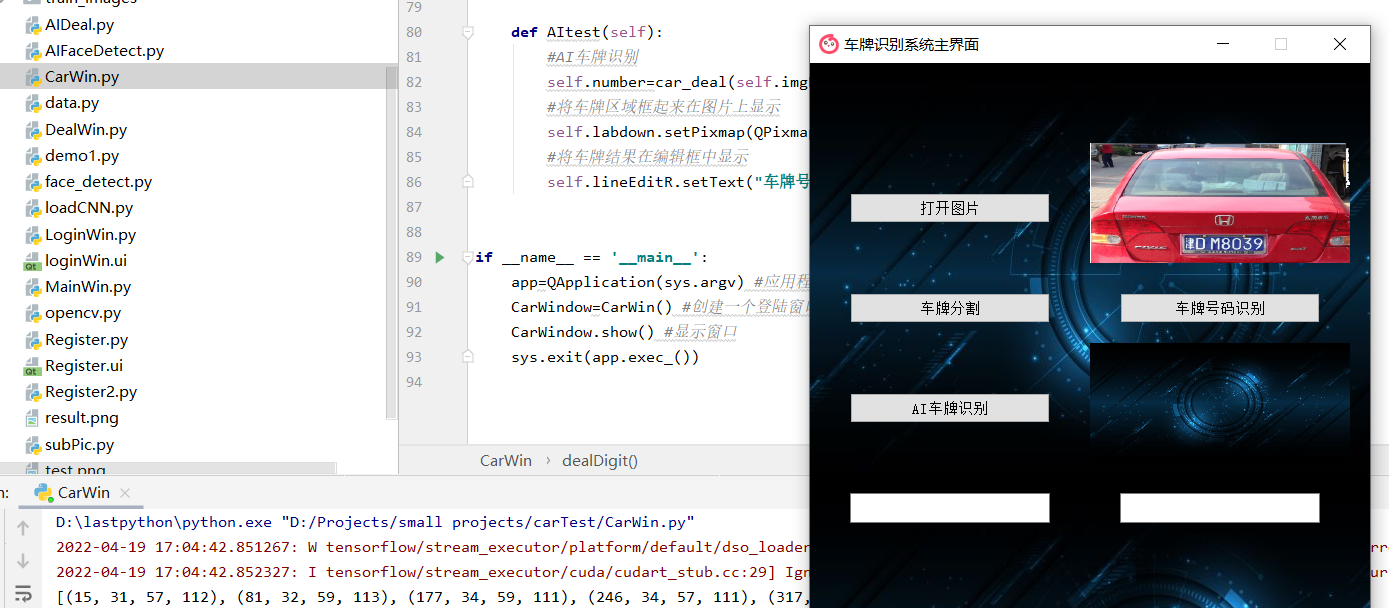``````车牌识别的几个步骤：

1.从图片上找到车牌的区域
2.从图片上截取车牌的区域
3.从这个车牌区域中分割出一个一个的字符图片，保存
4.字符图片挨个识别：先识别省份，再识别城市、再识别号码
5.三个部分合在一起，得到最终的车牌的详细信息

tensorflow:数据流编程 平台的工作方式：数据流图
1.创建图
2.给图中添加需要的模块
3.带入数据 进行计算 让数据在图中流动起来

1.特征提取
2.主要特征提取
3.主要特征汇总
4.分类汇总 预测属于哪一个类别（得到的都是概率）
``````

## 构建模型代码段：opencv.py

### 初始化参数

``````WIDTH=32 #设置图片的宽和高
HEIGHT=40
IMAGESIZE=1280 #32*40
interations=40 #训练次数
#2.给你的图片本应该是什么类别的 经过计算之后得出的结论是什么 该图片属于各种类别的概率 概率最大的就是识别出的类
NUM_CLASSES=34  #一张图片的分类可能是34种中的一种 最后要得出每一种的概率

#要识别的车牌号码的集合 34类输出
LETTER_NUM=('0','1','2','3','4','5','6','7','8','9',
'A','B','C','D','E','F','G','H','J','K','L','M',
'N','P','Q','R','S','T','U','V','W','X','Y','Z')
``````

### 输入层

``````#输入层：保存图片的信息 需要保存的地方 ---占位符
#x存特征数据   y存标签数据
x=tf.placeholder(tf.float32,shape=[None,IMAGESIZE]) #创建一个占位符 Shape第一个参数表示图片的数量(None表示多少张图片都可以 不做限制） 第二个参数是size
y=tf.placeholder(tf.float32,shape=[None,NUM_CLASSES]) #标签,规定了标签的数量

#修改一个图片的形状 保证每一张图片都是32*40
x_imgs=tf.reshape(x,[-1,WIDTH,HEIGHT,1]) #最后的1表示单通道 （灰度图） -1表示不限制有多少张图片 但是对宽度高度和单通道做了限制
``````

### 特征提取

``````#小部分每一个值的权重（tf.Variable()创建变量）
W_con1=tf.Variable(tf.random_normal([8,8,1,16],stddev=0.1),name='W_con1')  #小区域8*8,单通道深度为1，将深度从1变成16 stddev=0.1设置标准差 生成的随机数不会相差太大

#偏置
b_con1=tf.Variable(tf.constant(0.1,shape=),name='b_con1') #生成16个偏置 值都为0.1 #设置的偏置个数需要和输出的深度一致

#图片初始大小32*40*1
#卷积操作（区域乘以小区域对应的权重）

#激活函数
#把小于0的值用0替代 relu(0,x)取0和这个数的较大一方 【已经把小于0的那些无用内容剔除了---去除无效特征】 激活函数
jh_con1=tf.nn.relu(jj_con1+b_con1) #乘对应的权重并加上偏置后 relu把小于0的值用0替代

#池化
#主要特征提取---提取（均值 最大值）（一般都是2*2的区域) 取区域中的最大值 进行池化

#池化后图片的大小变为 （16*20）*16 在W_con1=tf.Variable()中将tf.random_normal的深度从1设置到16 因为池化区域和池化步长为（2 2） 所以相应的从32*40-》16*20
``````

### 再做一次卷积池化

``````#做第二次 第一次输出的深度是16 这次将深度从16变为32 且区域变为5*5
W_con2=tf.Variable(tf.random_normal([5,5,16,32],stddev=0.1),name='W_con1')
b_con2=tf.Variable(tf.constant(0.1,shape=),name='b_con2') #生成32个偏置【shape与输出的深度要同步】值都为0.1
jh_con2=tf.nn.relu(jj_con2+b_con2) #乘对应的权重并加上偏置后 relu把小于0的值用0替代
#图片的大小变为(16*20)*32
``````

### 全连接层

``````#图片数据转成1行 转为一维 从16*20*32转到512 这是全连接层？
W_fc1=tf.Variable(tf.random_normal([16*20*32,512],stddev=0.1),name="W_fc1") #权重
b_fc1=tf.Variable(tf.constant(0.1,shape=),name="b_fc1") #生成偏置 512个0.1
h_fc1_flat=tf.reshape(ch_con2,[-1,16*20*32]) #特征值 [-1,16*20*32] -1表示不管有多少张图片 但是每张图片大小要保证16*20*32
#采用 权重*特征值+偏置的 然后取最大值
h_fc1=tf.nn.relu(tf.matmul(h_fc1_flat,W_fc1)+b_fc1) #matmuL()注意要把权重放在右边
``````

### 删除部分神经元

``````#删除部分神经元---剩余多少神经元参与工作
keep_prob=tf.placeholder(tf.float32) #删除神经元的个数
h_fc1_drop=tf.nn.dropout(h_fc1,keep_prob)
``````

### 输出分类

``````#输出--分类 有NUM_CLASS个类
W_fc2=tf.Variable(tf.random_normal([512,NUM_CLASSES],stddev=0.1),name="W_fc2") #权重
b_fc2=tf.Variable(tf.constant(0.1,shape=[NUM_CLASSES]),name="b_fc2") #生成偏置
#直接计算结果
y_con=tf.matmul(h_fc1_drop,W_fc2)+b_fc2
``````

### 计算误差和精确率

``````
#网络搭建完成 接下来我们计算真实结果和识别出来结果的差距
#softmax_cross_entropy_with_logits 用来计算真实结果y和识别出来结果y_con的交叉熵
#reduce_mean 求均值 用均值来表示他们之间的差距
cross=tf.reduce_mean(tf.nn.softmax_cross_entropy_with_logits(labels=y,logits=y_con))

#只能看到损失值 不方便我们观察准确率
#准确率怎么表示---图片属于哪个分类 找最大值对应的下标 下标是几 就属于哪个分类
#tf.argmax(input,axis)根据axis取值的不同返回每行或者每列最大值的索引
#equal:bool类型的真和假 通过argmax其实就是从数组中挑出概率取到最大值的下标
#例：【20，15，30，40】 下标为3概率取到最大值 两者进行对比就可以判断预测值和真实值是否相同
"""

A = [[1,3,4,5,6]]
B = [[1,3,4,3,2]]

"""
correct=tf.equal(tf.argmax(y_con,1),tf.argmax(y,1))
#把bool类型转成数字类型 tf.cast进行数据类型的转换
accuracy=tf.reduce_mean(tf.cast(correct,tf.float32)) #准确率
``````

### 读取图片

``````#读取图片的函数
#这个函数的作用就是把读取到的每一张图片:图片特征数据和图片属于哪一个分类(标签数据)整合再一起 为模型训练准备好完整的数据集！

input_count = 0
for i in range(0, NUM_CLASSES):
dir = './train_images/training-set/%s/' % str(i)  # 这里可以改成你自己的图片目录，i为分类标签
for rt, dirs, files in os.walk(dir):
for filename in files:
input_count += 1

# 定义对应维数和各维长度的数组
# input_images特征数据
input_images = np.array([ * IMAGESIZE for i in range(input_count)])
# 标签数据：
input_labels = np.array([ * NUM_CLASSES for i in range(input_count)])

# 第二次遍历图片目录是为了生成图片数据和标签
index = 0
for i in range(0, NUM_CLASSES):
dir = './train_images/training-set/%s/' % str(i)  # 这里可以改成你自己的图片目录，i为分类标签
for rt, dirs, files in os.walk(dir):
for filename in files:
filename = dir + filename
img = Image.open(filename)
width = img.size
height = img.size
for h in range(0, height):
for w in range(0, width):
# 通过这样的处理，使数字的线条变细，有利于提高识别准确率
if img.getpixel((w, h)) > 230:
input_images[index][w + h * width] = 0
else:
input_images[index][w + h * width] = 1
input_labels[index][i] = 1
index += 1

# 第一次遍历图片目录是为了获取图片总数
val_count = 0
for i in range(0, NUM_CLASSES):
dir = './train_images/training-set/%s/' % str(i)  # 这里可以改成你自己的图片目录，i为分类标签
for rt, dirs, files in os.walk(dir):
for filename in files:
val_count += 1

# 定义对应维数和各维长度的数组
val_images = np.array([ * IMAGESIZE for i in range(val_count)])
val_labels = np.array([ * NUM_CLASSES for i in range(val_count)])

# 第二次遍历图片目录是为了生成图片数据和标签
index = 0
for i in range(0, NUM_CLASSES):
dir = './train_images/training-set/%s/' % str(i)  # 这里可以改成你自己的图片目录，i为分类标签
for rt, dirs, files in os.walk(dir):
for filename in files:
filename = dir + filename

img=Image.open(filename)
width = img.size
height = img.size
for h in range(0, height):
for w in range(0, width):
# 通过这样的处理，使数字的线条变细，有利于提高识别准确率
if img.getpixel((w, h)) > 230:
val_images[index][w + h * width] = 0
else:
val_images[index][w + h * width] = 1
val_labels[index][i] = 1
index += 1
# 返回图片的特征数据，标签数据以及图片张数
return input_images, input_labels, input_count, val_images, val_labels, val_count
``````

### 模型的训练与保存

``````
#启动图 把数据带入 让数据在图中流动
#怎么启动图：
with tf.Session() as sess: #Session提供了Operation执行和Tensor求值的环境;
#怎么执行初始化
sess.run(init)
# 要训练 需要数据--大量的图片数据 需要读取每一张图片
input_images, input_labels, input_count, val_images, val_labels, val_count=picRead_pre()
# input_images:x 输入, input_labels:y 输出, input_count 数据总数
#开始训练 不要一下子全部给他 只给一部分 分块给他
#每一次给60张图片 input_count表示图片的总数
batch_size=60 #分组大小设置为60
batches_count=int(input_count/batch_size) #分组的数量
res=input_count%batch_size #最后一次训练 使用剩余图片

#训练次数：100次
for i in range(interations): #训练次数
for n in range(batches_count): #一次完整的图片全部训练需要做几次
#怎么把数据带入图中 [0,60) [60,120) 每次喂入一组数据
sess.run(train_step,feed_dict={x:input_images[n*batch_size:(n+1)*batch_size],
y:input_labels[n*batch_size:(n+1)*batch_size],
keep_prob:0.5}) #只给一半的神经元
if res>0: #如果图片剩余
start_index=batches_count*batch_size #整数组的最后一个为hi
sess.run(train_step,feed_dict={x:input_images[start_index:input_count-1],
y:input_labels[start_index:input_count-1],
keep_prob:0.5})

#每做五次 打印一次结果
if i%1==0:
#得到准确率
accry=sess.run(accuracy,feed_dict={x:val_images,
y:val_labels,
keep_prob:1.0}) #给全部的神经元

print("第 %d 次训练 准确率为 %0.5f%%"%(i,accry*100))

print("训练完成")

#模型的保存
saver=tf.train.Saver()
saver.save(sess,"./model/letter_digits_model.ckpt")

#模型保存完了 怎么使用呢
#加载模型 首先必须构建一个一模一样的网络
``````

``````#使用模型
#1.构建一模一样的网络
#2.启动图
#3.读取一张图片进行预测
#4.将图片数据存储到img_data中
#5.把数据带入计算结果

#模型使用的流程：1.构建模型 2.训练模型 3.保存模型 4.加载模型（构建一个一模一样的模型） 5.给图片进行预测 6.结果对比
import os
import numpy as np
import tensorflow as tf
from PIL import Image

WIDTH=32
HEIGHT=40
IMAGESIZE=1280 #32*40

#2.给你的图片本应该是什么类别的 经过计算之后得出的结论是什么 该图片属于各种类别的概率 概率最大的就是识别出的类
NUM_CLASSES=34  #一张图片的分类可能是34种中的一种 最后要得出每一种的概率

#要识别的车牌号码的集合 34类输出
LETTER_NUM=('0','1','2','3','4','5','6','7','8','9',
'A','B','C','D','E','F','G','H','J','K','L','M',
'N','P','Q','R','S','T','U','V','W','X','Y','Z')

#输入层：保存图片的信息 需要保存的地方 ---占位符
#x存特征数据   y存标签数据
x=tf.placeholder(tf.float32,shape=[None,IMAGESIZE]) #创建一个占位符 Shape第一个参数表示图片的数量(None表示多少张图片都可以 不做限制） 第二个参数是size
y=tf.placeholder(tf.float32,shape=[None,NUM_CLASSES]) #标签,规定了标签的数量

#修改一个图片的形状 保证每一张图片都是32*40
x_imgs=tf.reshape(x,[-1,WIDTH,HEIGHT,1]) #最后的1表示单通道 （灰度图） -1表示不限制有多少张图片 但是对宽度高度和单通道做了限制

#特征提取：卷积层实现--提取图像中每一个小部分的特征 自己约定 暂时定为8*8
#小部分每一个值的权重（tf.Variable()创建变量）
W_con1=tf.Variable(tf.random_normal([8,8,1,16],stddev=0.1),name='W_con1')  #小区域8*8,单通道深度为1，将深度从1变成16 stddev=0.1设置标准差 生成的随机数不会相差太大

#偏置
b_con1=tf.Variable(tf.constant(0.1,shape=),name='b_con1') #生成16个偏置 值都为0.1 #设置的偏置个数需要和输出的深度一致

#图片初始大小32*40*1
#卷积操作（区域乘以小区域对应的权重）

#激活函数
#把小于0的值用0替代 relu(0,x)取0和这个数的较大一方 【已经把小于0的那些无用内容剔除了---去除无效特征】 激活函数
jh_con1=tf.nn.relu(jj_con1+b_con1) #乘对应的权重并加上偏置后 relu把小于0的值用0替代

#池化
#主要特征提取---提取（均值 最大值）（一般都是2*2的区域) 取区域中的最大值 进行池化

#池化后图片的大小变为 （16*20）*16 在W_con1=tf.Variable()中将tf.random_normal的深度从1设置到16 因为池化区域和池化步长为（2 2） 所以相应的从32*40-》16*20

#做第二次 第一次输出的深度是16 这次将深度从16变为32 且区域变为5*5
W_con2=tf.Variable(tf.random_normal([5,5,16,32],stddev=0.1),name='W_con1')
b_con2=tf.Variable(tf.constant(0.1,shape=),name='b_con2') #生成32个偏置【shape与输出的深度要同步】值都为0.1
jh_con2=tf.nn.relu(jj_con2+b_con2) #乘对应的权重并加上偏置后 relu把小于0的值用0替代
#图片的大小变为(16*20)*32

#图片数据转成1行 转为一维 从16*20*32转到512 这是全连接层？
W_fc1=tf.Variable(tf.random_normal([16*20*32,512],stddev=0.1),name="W_fc1") #权重
b_fc1=tf.Variable(tf.constant(0.1,shape=),name="b_fc1") #生成偏置 512个0.1
h_fc1_flat=tf.reshape(ch_con2,[-1,16*20*32]) #特征值 [-1,16*20*32] -1表示不管有多少张图片 但是每张图片大小要保证16*20*32
#采用 权重*特征值+偏置的 然后取最大值
h_fc1=tf.nn.relu(tf.matmul(h_fc1_flat,W_fc1)+b_fc1) #matmuL()注意要把权重放在右边

#删除部分神经元---剩余多少神经元参与工作
keep_prob=tf.placeholder(tf.float32) #删除神经元的个数
h_fc1_drop=tf.nn.dropout(h_fc1,keep_prob)

#输出--分类 有NUM_CLASS个类
W_fc2=tf.Variable(tf.random_normal([512,NUM_CLASSES],stddev=0.1),name="W_fc2") #权重
b_fc2=tf.Variable(tf.constant(0.1,shape=[NUM_CLASSES]),name="b_fc2") #生成偏置
#直接计算结果
y_con=tf.nn.softmax(tf.matmul(h_fc1_drop,W_fc2)+b_fc2)
``````

``````
init=tf.global_variables_initializer()  #变量初始化

with tf.Session() as sess:
sess.run(init)
#加载保存好的模型
saver=tf.train.Saver()
saver.restore(sess,"./model/letter_digits_model.ckpt")

#开始使用模型进行预测
#给图片--读图片
licence_num="" #用于拼接车牌号码
for i in range(3,8): #这里是根据车牌分割之后 result文件夹下面的图片来读取的
path="./result/imgs%d.jpg"%(i) #%d
img=Image.open(path)

width=img.size
height=img.size

#创建一个空的二维数组 保存图片中的相关信息(图片宽度和高度的信息）
img_data=[*IMAGESIZE for i in range(1)]

for h in range(0,height):
for w in range(0,width):
#图片是黑白的 因此对像素点进行拆分 将拆分的结果表示成1和0两种情况
if img.getpixel((w,h))max: #[[]] 被嵌套了 所以先用取一下
max=result[j]
max_index=j
#把每一张图片识别出来的数符加到末尾
licence_num=licence_num+LETTER_NUM[max_index] #把各个字母数字拼接起来
print("车牌号码:%s"%licence_num)
return licence_num
``````

### 当然都懂大家想走捷径的心哈哈哈哈 完整的项目工程文件可以私信我 一杯奶茶的钱(15r) 大家少走些弯路 希望大家都可以快速完成自己的项目！(应付作业 狗头）

（项目安装包）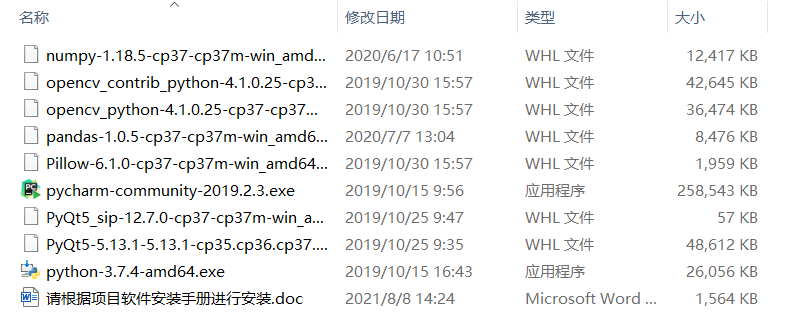（项目文件）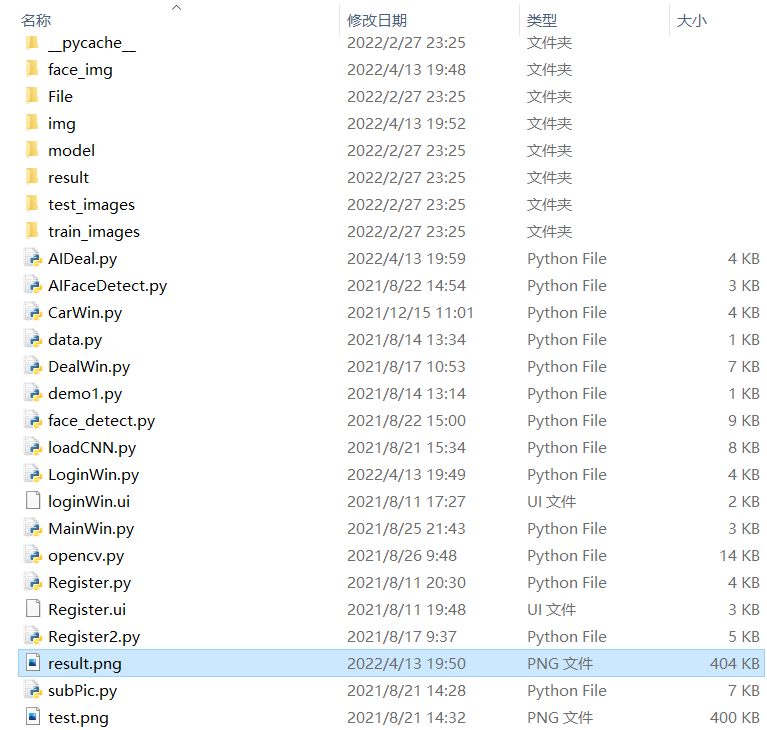（车牌识别界面）（使用上述 AI车牌识别按钮需要将AIDeal.py文件修改问自己的百度API，可以参照我主页的另一篇博客 也可以直接使用 车牌号码识别按钮调用已经训练好的模型）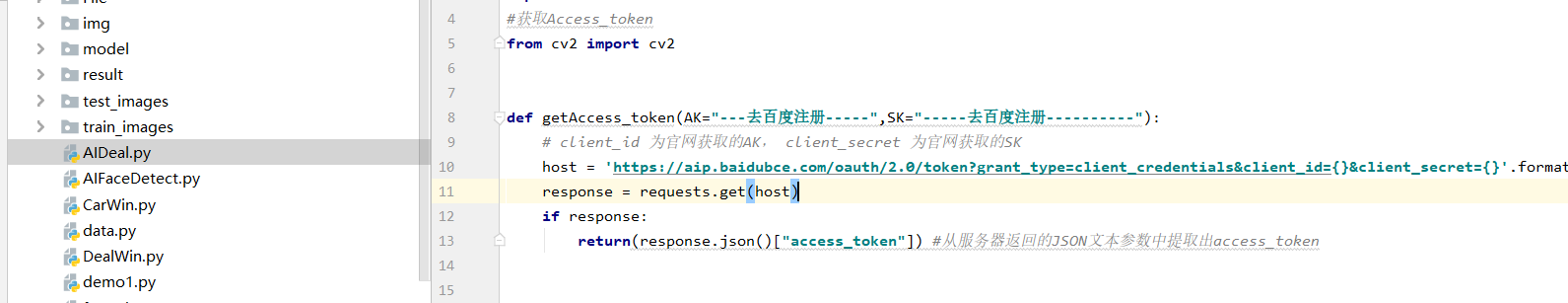（项目文件中还包含当时写的一些其它UI 可以自己取舍）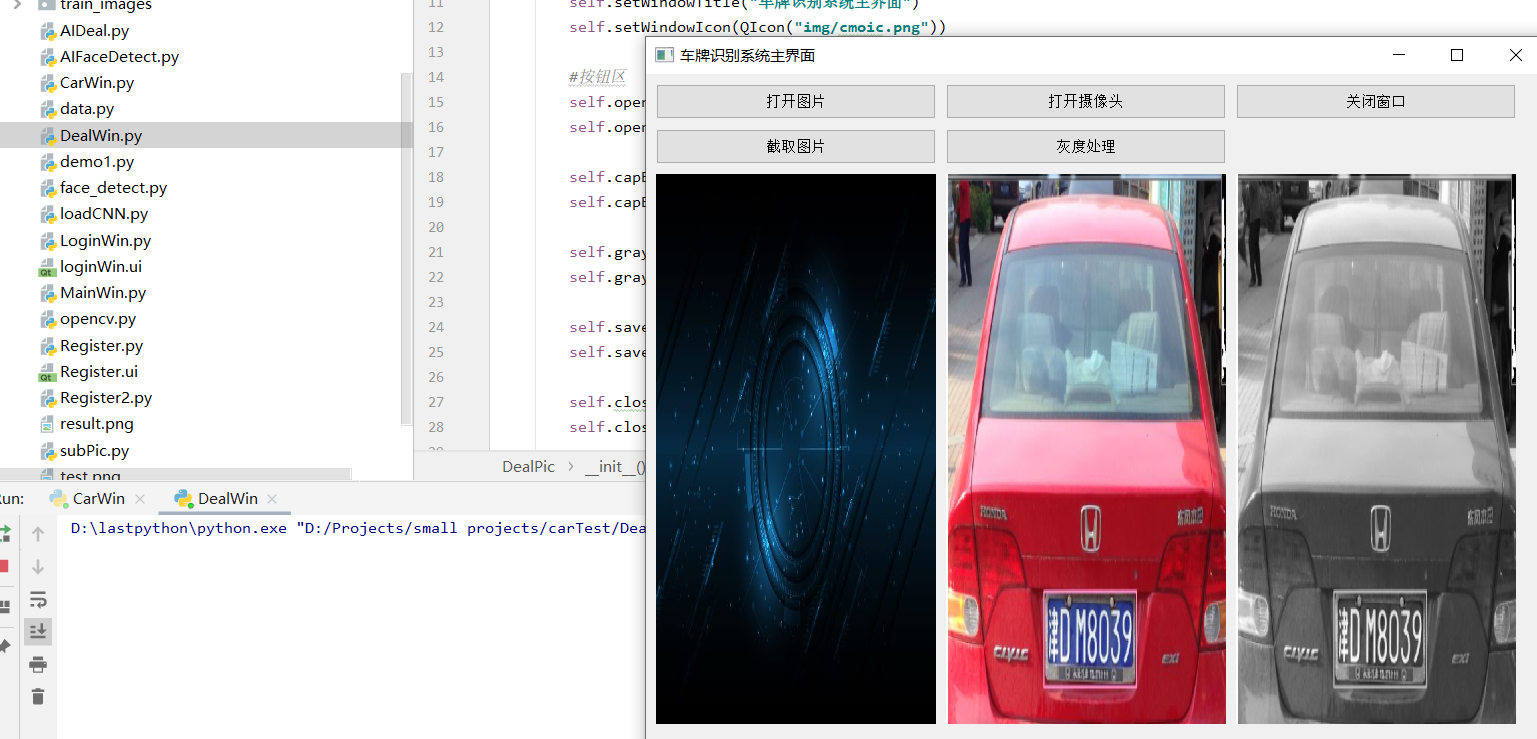（当时写着玩的人脸识别与颜值打分系统也留在文件里 )（手动狗头） 需要把这里换成自己的百度API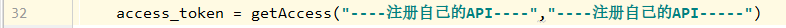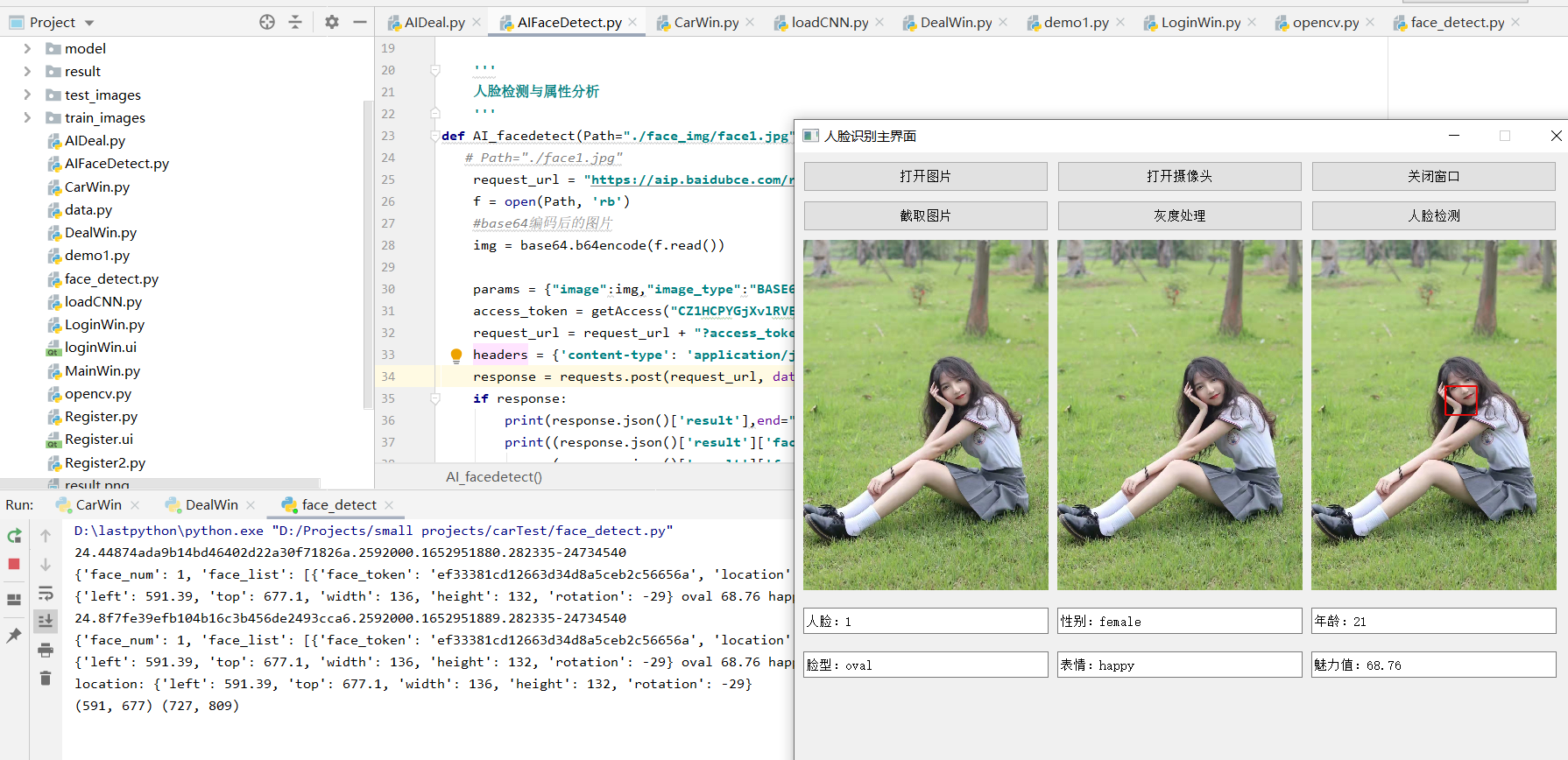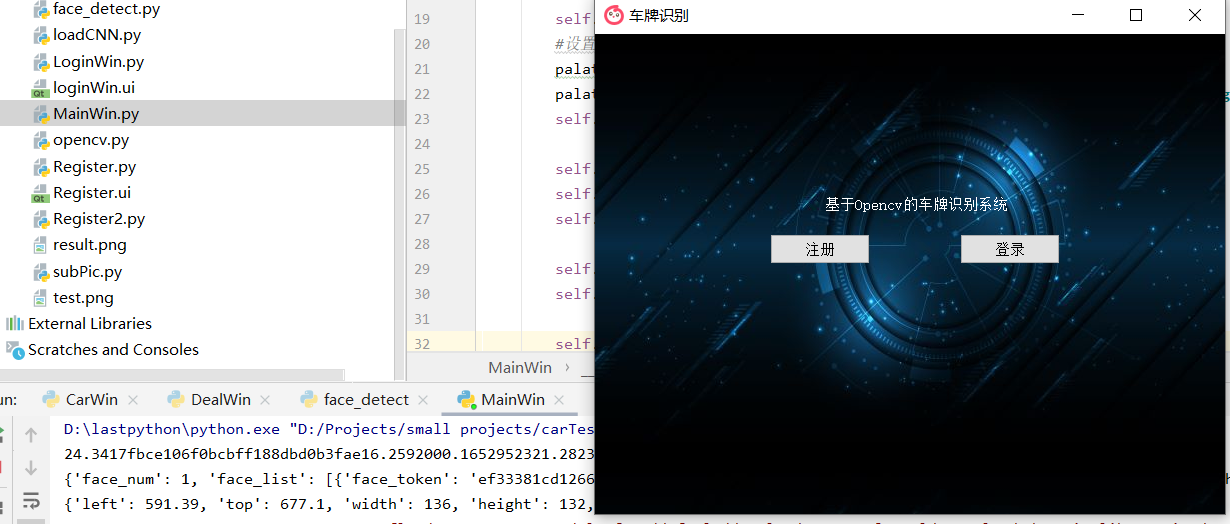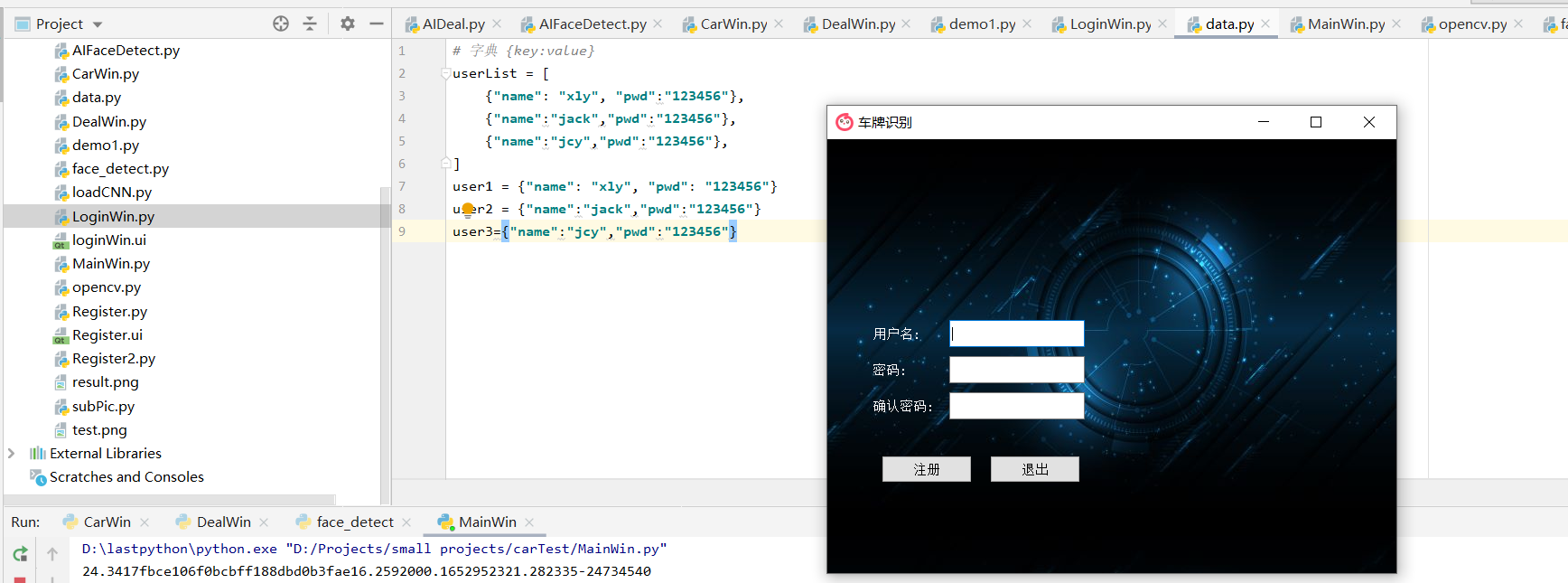（还可以提供简单的项目答疑哦）Original: https://blog.csdn.net/SOBE_rrr/article/details/119924079
Author: SOBE_rrr
Title: 利用CNN卷积神经网络实现车牌识别（tensorflow）附完整Python项目代码

## Title: PanoSim 5 功能模块简介--Sensor Builder

4.3 SensorBuilder

SensorBuilder 是用来给所选车辆添加传感器的模块。本章介绍该模块的操作界面，关于传感器模型参数的具体介绍，请参看 5.4 传感器模型。双击 PanoSim5 安装包中的图标或点击 Experiment GUI 界面提供的快捷接 口"Tools-SensorBuilder"可以启动 PanoSim SensorBuilder GUI 界面。SensorBuilder 启动时显 示以下界面，传感器主界面功能分区如图 4.30 所示。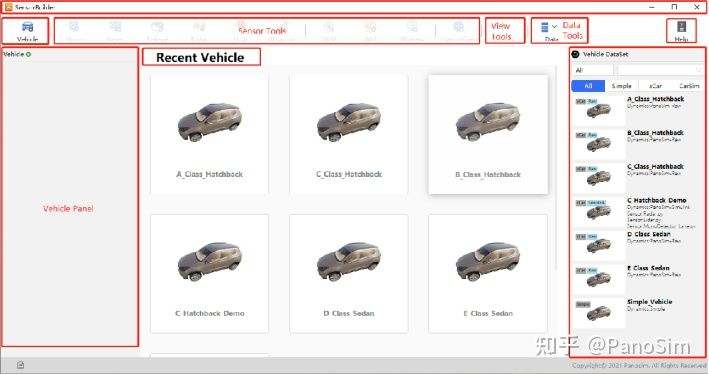4.3.1 工具栏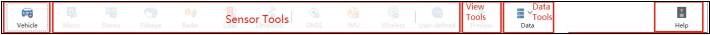4.3.1.1 Sensor Tools

SensorBuilder 主要用于被测车辆和被测车辆所搭载传感器的设置，可以显示所有可用 的被测车辆和传感器列表，能够加载被测车辆模型并显示传感器感知范围，完成为被测车 辆添加传感器、编辑传感器内外部参数等操作。

（1）Vehicle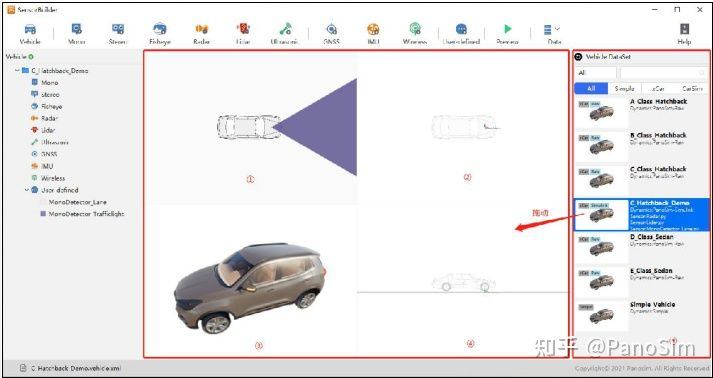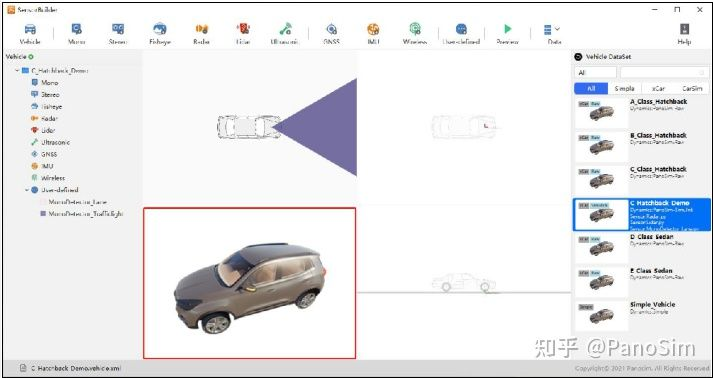（2）Sensor Tools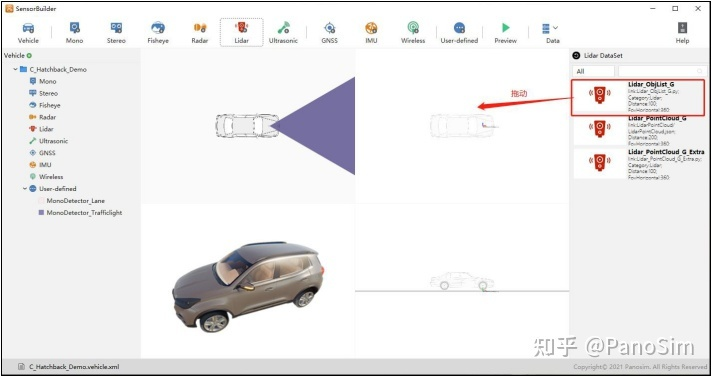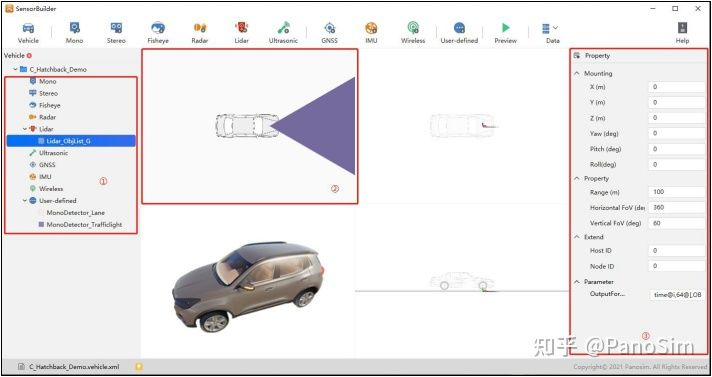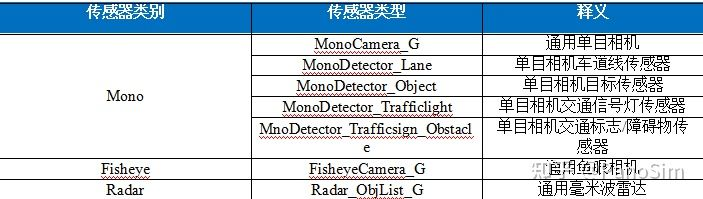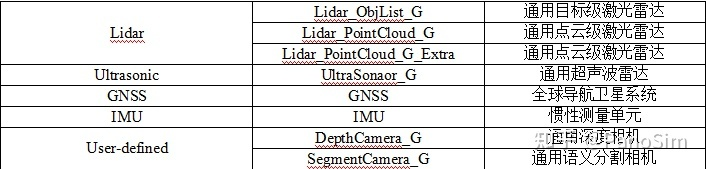4.3.1.2 View Tools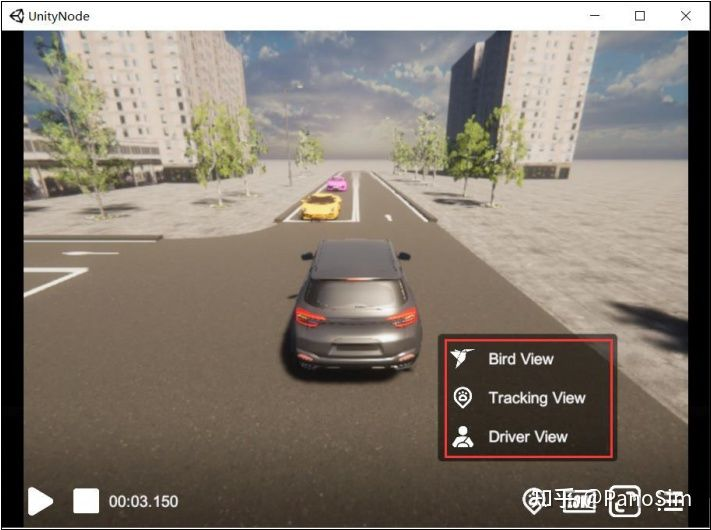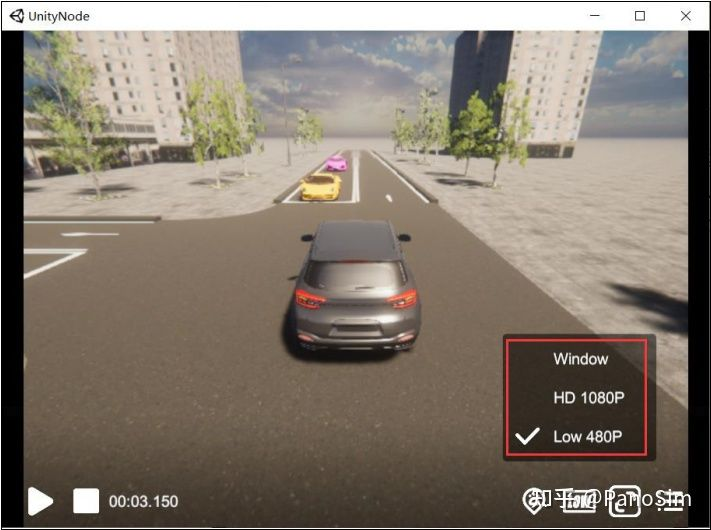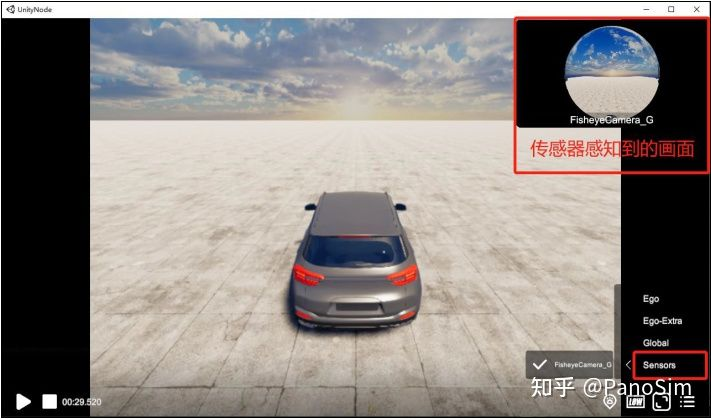4.3.1.3 Data Tools

4.3.1.4 Help Support

4.3.2 Sensor 配置窗口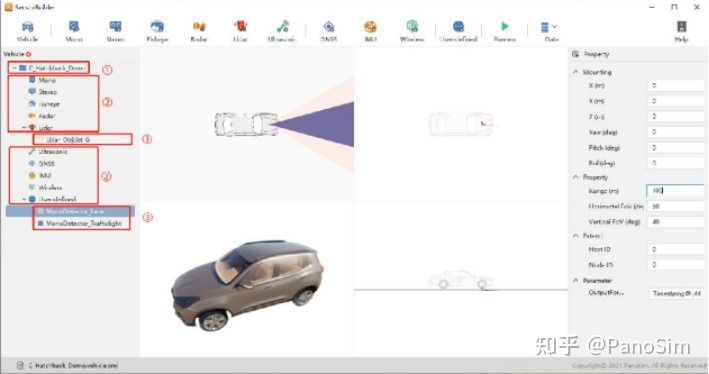4.3.3 工作区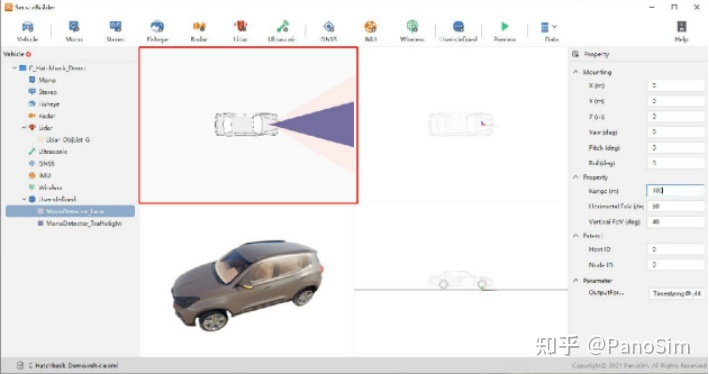4.3.4 属性栏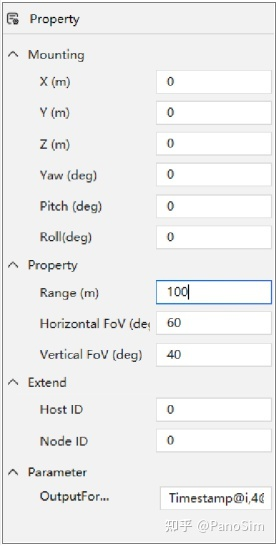### 4.3.5 状态栏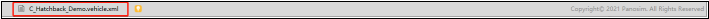Original: https://blog.csdn.net/PanoSim666/article/details/126227761
Author: PanoSim666
Title: PanoSim 5 功能模块简介--Sensor Builder